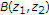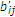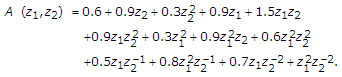# Erratum to The PLSI Method of Stabilizing Two-Dimensional Nonsymmetric Half-Plane Recursive Digital Filters

The Original Article was published on 18 August 2003

## Erratum

The authors would like to bring to the kind attention of the readers the following corrections to the paper .

Equation (23) in page number 917 is as follows:(1)

It should be noted that in (23) and all equations in the rest of the paper that contain the term, this term is the constant term of the polynomial, which is the stable version of the 2D PLSI polynomial. Since we have assumed that's are the coefficients of the 2D PLSI polynomial, the coefficients of the polynomialare's.

Equation (46) in page number 920 is as follows:(2)

This equation may be corrected as follows:(3)

## References

1. Gangatharan N, Reddy PS: The PLSI method of stabilizing two-dimensional nonsymmetric half-plane recursive digital filters. EURASIP Journal on Applied Signal Processing 2003, 2003(9):914-921.

## Acknowledgments

We would like to thank K. R. Santhi, Department of Computer Engineering and Information Technology, Faculty of Engineering, Kigali Institute of Science and Technology (KIST), Rwanda, for bringing to our attention one of the above corrections.

## Author information

Authors

### Corresponding author

Correspondence to N. Gangatharan.

The online version of the original article can be found at 10.1155/S1110865703303099

## Rights and permissions

Reprints and Permissions

Gangatharan, N., Reddy, P.S. Erratum to The PLSI Method of Stabilizing Two-Dimensional Nonsymmetric Half-Plane Recursive Digital Filters. EURASIP J. Adv. Signal Process. 2008, 647075 (2007). https://doi.org/10.1155/2008/647075